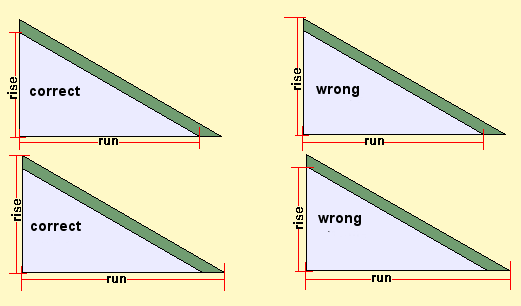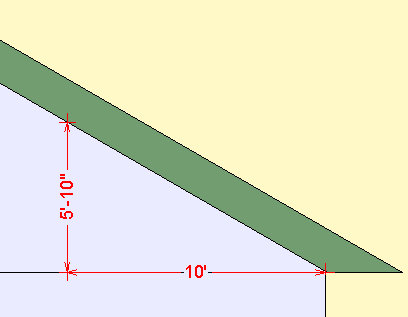Spike's Calculators

# Roof Pitch Calculator

Calculate the pitch of a roof from the rise and rune measurements.

Measure a horizontal distance (run) inward from the edge of the building either on top of the fascia board or below. Just make sure you measure the vertical height (rise) to the same as you did the run measurement, to the top of the fascia or the bottom.The run measurement can be any length, it does not have to go to the centre. A longer measurement might make it a bit easier or more accurate.#### Example:

You measured 10 feet in (horizontal) from the outside wall. At that point you measure the rise measurement perpendicular (at a right angle) to the run measurement. In this case it is 5 feet 10 inches.

##### The measurements will have to be converted to the same units.
```10' = 120 inches
5'-10" = 70 inches```
##### Pitch in 12 : ( rise ÷ run * 12)
```70  ÷ 120 = 0.5833333333333333 * 12 =  7
7 : 12 pitch```
##### Grade in a percentage: (rise ÷ run * 100)
```70  ÷ 120 = 0.5833333333333333 * 100 = 58.3 (rounded to one digit)
grade of the roof  = 58.3%```
##### Slope in degrees: Arctan ( rise ÷ run )
```Arctan ( 0.583) =  30.2421853687
roof angle = 30.3° (rounded to one digit)```
##### And as an additional bonus:
use the secant trig function on your calculator and plug in the roof angle of 30.25642291379965° and the result of 1.1577 is the slope factor for this roof which can be used for calculating the length of the rafters and the square footage of the roof.

### Roof Pitch from Rise and Run

Run ft in
Rise ft in

#### Results:

 Roof Pitch :12 Grade % Roof Angle °

#### Calculation

1. enter the horizontal, the run length in foot and inch
2. enter the vertical, the rise length in foot and inch

#### Results

1. the amount the roof rises in a run of 12 inches
2. the slope (grade) of the roof in a percentage
3. the angle of the roof in degrees